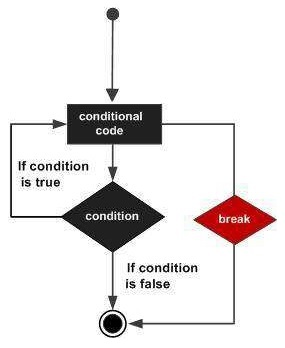# Swift - Break Statement

The break statement in C programming language has the following two usages −

• When a break statement is encountered inside a loop, the loop is immediately terminated and the program control resumes at the next statement following the loop.

• It can be used to terminate a case in switch statement (covered in the next chapter).

If you are using nested loops (i.e., one loop inside another loop), then the break statement will stop the execution of the innermost loop and start executing the next line of the code after the block.

## Syntax

The syntax for a break statement in Swift 4 is as follows −

```break
```

## Flow Diagram## Example

```var index = 10

repeat {
index = index + 1
if( index == 15 ){
break
}
print( "Value of index is \(index)")
} while index < 20
```

When the above code is compiled and executed, it produces the following result −

```Value of index is 11
Value of index is 12
Value of index is 13
Value of index is 14
```
swift_loops.htm